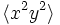# Hereditarily characteristic not implies cyclic in finite

This article gives the statement and possibly, proof, of a non-implication relation between two subgroup properties, when the big group is a finite group. That is, it states that in a finite group, every subgroup satisfying the first subgroup property (i.e., hereditarily characteristic subgroup) need not satisfy the second subgroup property (i.e., cyclic characteristic subgroup)
View all subgroup property non-implications | View all subgroup property implications

## Statement

It is possible to have a finite group$G$, and a subgroup$K$ of$H$ such that:

1.$K$ is a Hereditarily characteristic subgroup (?) of$G$: For every subgroup$H$ of$K$,$H$ is a characteristic subgroup of$G$.
2.$K$ is not a cyclic group.

## Proof

Consider the semidirect product of cyclic group:Z4 and cyclic group:Z4 where the generator of the acting group acts via the inverse map on the other group. In other words, it has the presentation:$G := \langle x,y \mid x^4 = y^4 = e, yxy^{-1} = x^3 \rangle$.

Let$K$ be the subgroup$\langle x^2, y^2 \rangle$. Then,$K$ is a Klein four-group but every subgroup of$K$ is characteristic in$G$. We explain here why each of the subgroups of order two is characteristic:

• The subgroup$\langle x^2 \rangle$ is the derived subgroup.
• The subgroup$\langle y^2 \rangle$ is the subgroup generated by the unique element that is a square but not a commutator.
• The subgroup$\langle x^2y^2 \rangle$ is the subgroup generated by the unique element that is a product of squares but not a square.$K$ itself is$\mho^1(G)$ and is characteristic in$G$, and the trivial subgroup is characteristic in$G$. Thus, all subgroups of$K$ are characteristic in$G$.